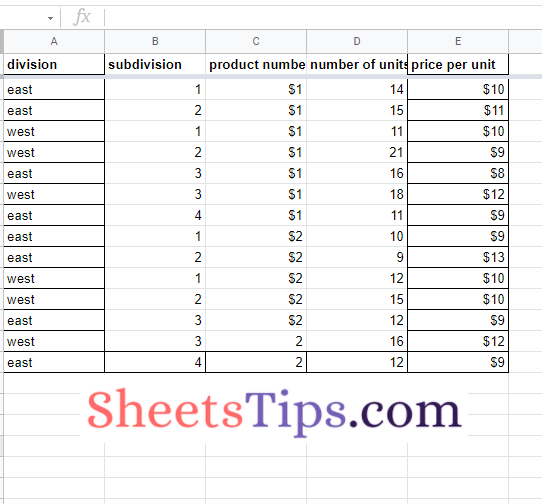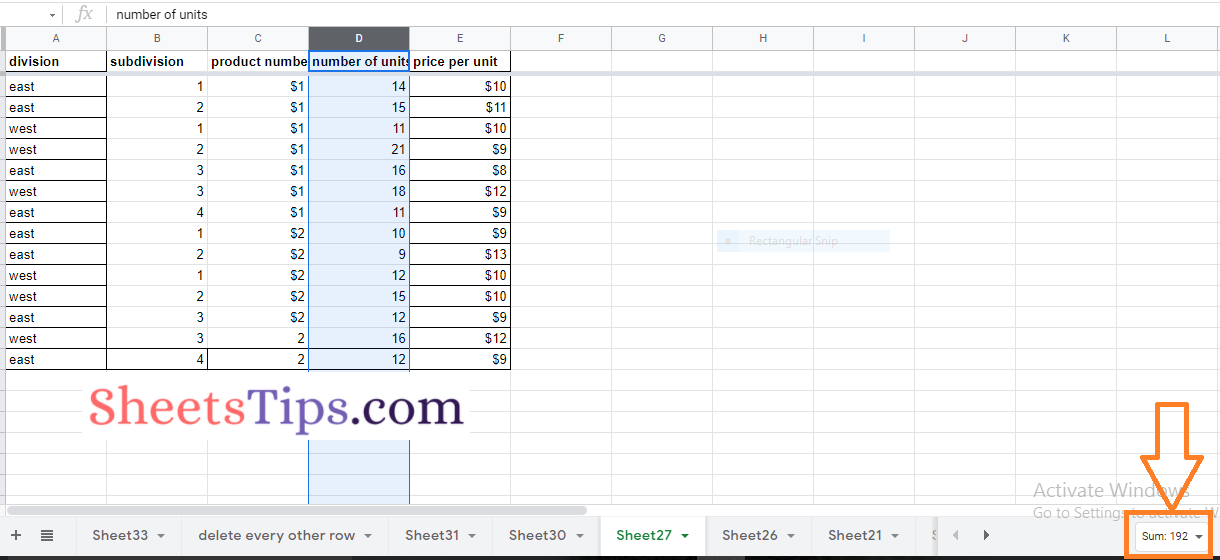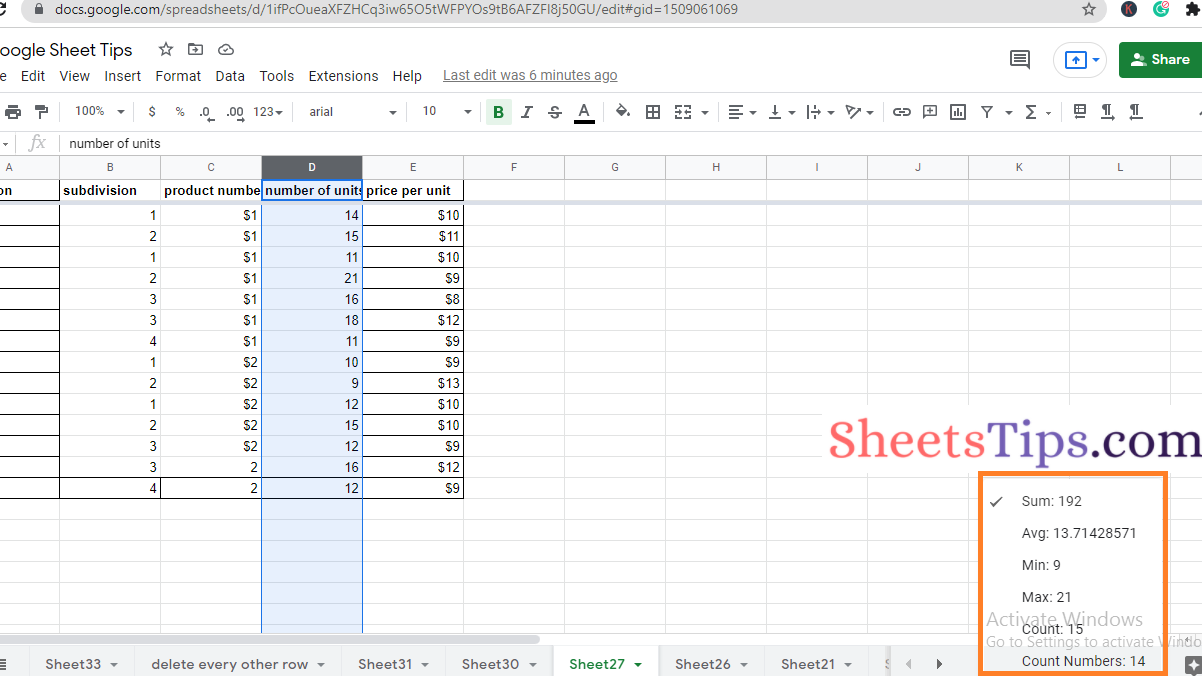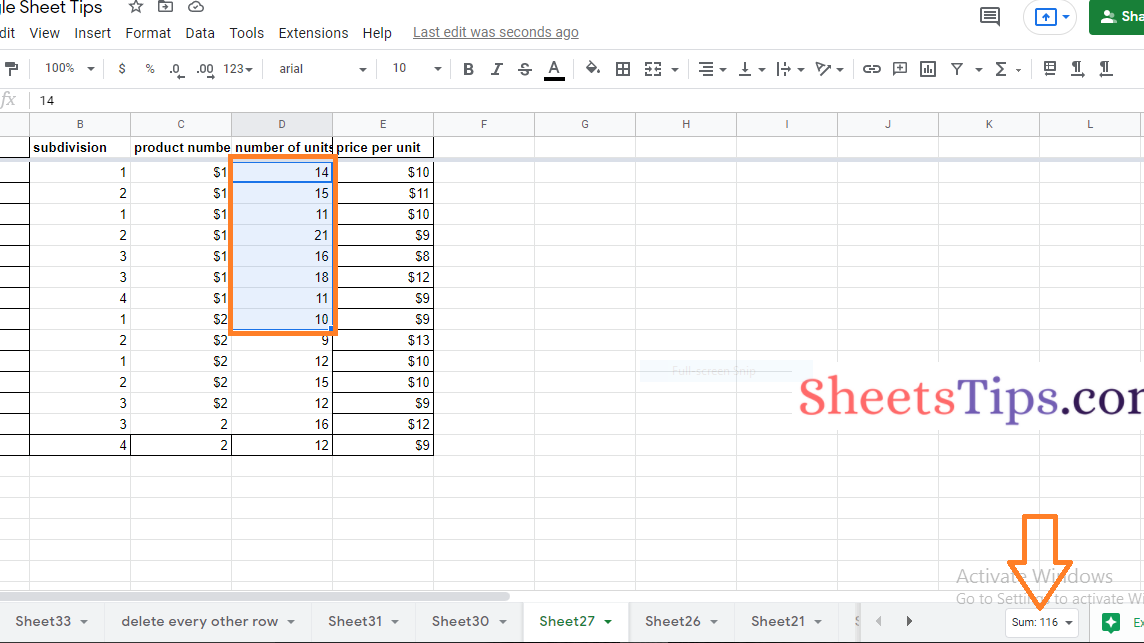# How to See Basic Calculations Without Formulas in Google Sheets?

At times, when we are using Google Sheets we might fall under a situation where we would like to view the sum or average of the numbers without applying formulas. Google Sheets allows us to count, minimum value, maximum value, the sum of the numbers, average numbers, count numbers, and so on without applying formulas. In this article, let us understand how to see basic calculations without formulas in Spreadsheet using Google Sheets Tips provided on this page. Read further to find more.## List of Calculations Available in Google Sheets without Formulas

We can perform various calculations in Google Sheets without using formulas and they are as follows:

1. Sum
2. Average
3. Count
4. Count Number
5. Minimum Number
6. Maximum Number

### How to Perform Calculations Without Formulas?

Consider the following dataset which has sales reports region-wise.• Step 2: Move to the Sheet which has a dataset where you want to perform basic calculations without formulas.
• Step 3: Now select the column or row where you want to perform the basic mathematical calcutions.• Step 4: As soon as you select the column, you will be provided with various options towards the footer of the Google Spreadsheet as shown below.
• Step 5: Now to add the total numbers in a column, choose “Sum” from the drop down menu.
• Step 6: To find the Average of the number, choose “Avg” from the drop down menu.
• Step 7: To find the Minium number in the list of numbers, choose “Min” from the drop down menu.
• Step 8: To find the Maximum number in the number list, choose “Max” from the drop down menu.
• Step 9: To find the count of the dataset, you can choose “Count” in the drop down list.
• Step 10: To count only numbers, excluding the dataset, you can choose “Count Numbers” from the drop-down list.### How To Perform Basic Calculations without Applying Formulas for Specified Cells?

In the previous section, we discussed how to calculate numbers without applying formulas in Google Spreadsheet for the entire column or row. To perform calculations without using formulas only for the specified cells are as follows: